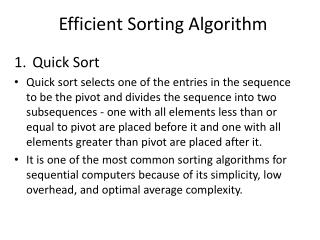DownloadDownload PresentationEfficient Sorting Algorithm

# Efficient Sorting Algorithm

Download Presentation## Efficient Sorting Algorithm

- - - - - - - - - - - - - - - - - - - - - - - - - - - E N D - - - - - - - - - - - - - - - - - - - - - - - - - - -
##### Presentation Transcript

1. Efficient Sorting Algorithm Quick Sort Quick sort selects one of the entries in the sequence to be the pivot and divides the sequence into two subsequences - one with all elements less than or equal to pivot are placed before it and one with all elements greater than pivot are placed after it. It is one of the most common sorting algorithms for sequential computers because of its simplicity, low overhead, and optimal average complexity.

2. Algorithm steps : • Choose a pivot value. Take the value of the middle element as pivot value, but it can be any value, which is in range of sorted values, even if it doesn't present in the array. • Partition. Rearrange elements in such a way, that all elements which are lesser than the pivot go to the left part of the array and all elements greater than the pivot, go to the right part of the array. Values equal to the pivot can stay in any part of the array. Notice that array may be divided in to non-equal parts. • Sort both parts. Apply quicksort algorithm recursively to the left and the right parts of the array.

3. Example